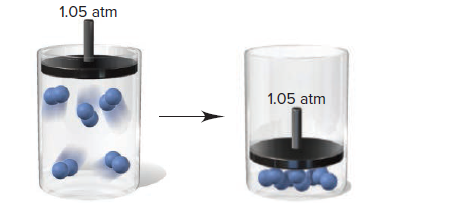# Problem: The scene below represents a physical change taking place in a piston-cylinder assembly:Can you determine whether ΔEsurr is +, −, or 0? Explain.

###### FREE Expert Solution

$\overline{)\mathbf{∆}\mathbf{E}\mathbf{=}\mathbf{q}\mathbf{+}\mathbf{w}}$

For heat, q:

+q → if the system gains, takes in, or absorbs heat or energy
–q → if the system loses, evolves, gives off, or releases heat or energy

For work, w:

+w → work is done on the system by the surroundings (volume: compresses)
–w → work is done by the system on the surroundings (volume: expands)

Given:

(assuming the physical change is from gas to solid)

heat is removed going from gas to liquid

82% (322 ratings)###### Problem Details

The scene below represents a physical change taking place in a piston-cylinder assembly:

Can you determine whether ΔEsurr is +, −, or 0? Explain.Frequently Asked Questions

What scientific concept do you need to know in order to solve this problem?

Our tutors have indicated that to solve this problem you will need to apply the Internal Energy concept. You can view video lessons to learn Internal Energy. Or if you need more Internal Energy practice, you can also practice Internal Energy practice problems.

What professor is this problem relevant for?

Based on our data, we think this problem is relevant for Professor Islam's class at UIC.

What textbook is this problem found in?

Our data indicates that this problem or a close variation was asked in Chemistry: The Molecular Nature of Matter and Change - Silberberg 8th Edition. You can also practice Chemistry: The Molecular Nature of Matter and Change - Silberberg 8th Edition practice problems.